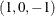Model Statement Usage

MODEL <transform(dependents </ t-options>)><transform(dependents </ t-options>)=> transform(independents </ t-options>)<transform(independents </ t-options>)…> </ a-options> ;

Here are some examples of model statements:

• linear regression

model identity(y) = identity(x);

• a linear model with a nonlinear regression function

model identity(y) = spline(x / nknots=5);

• multiple regression

model identity(y) = identity(x1-x5);

• multiple regression with nonlinear transformations

model spline(y / nknots=3) = spline(x1-x5 / nknots=3);

• multiple regression with nonlinear but monotone transformations

model mspline(y / nknots=3) = mspline(x1-x5 / nknots=3);

• multivariate multiple regression

model identity(y1-y4) = identity(x1-x5);

• canonical correlation

model identity(y1-y4) = identity(x1-x5) / method=canals;

• redundancy analysis

model identity(y1-y4) = identity(x1-x5) / method=redundancy;

• preference mapping, vector model (Carroll, 1972)

model identity(Attrib1-Attrib3) = identity(Dim1-Dim2);

• preference mapping, ideal point model (Carroll, 1972)

model identity(Attrib1-Attrib3) = point(Dim1-Dim2);

• preference mapping, ideal point model, elliptical (Carroll, 1972)

model identity(Attrib1-Attrib3) = epoint(Dim1-Dim2);

• preference mapping, ideal point model, quadratic (Carroll, 1972)

model identity(Attrib1-Attrib3) = qpoint(Dim1-Dim2);

• metric conjoint analysis

model identity(Subj1-Subj50) = class(a b c d e f / zero=sum);

• nonmetric conjoint analysis

model monotone(Subj1-Subj50) = class(a b c d e f / zero=sum);

• main effects, two-way interaction

model identity(y) = class(a|b);

• less-than-full-rank model—main effects and two-way interaction are constrained to sum to zero

model identity(y) = class(a|b / zero=sum);

• main effects and all two-way interactions

model identity(y) = class(a|b|c@2);

• main effects and all two- and three-way interactions

model identity(y) = class(a|b|c);

• main effects and only the b*c two-way interaction

model identity(y) = class(a b c b*c);

• seven main effects, three two-way interactions

model identity(y) = class(a b c d e f g a*b a*c a*d);

• deviations-from-means (effects or) coding, with an a reference level of ’1’ and a b reference level of ’2’

model identity(y) = class(a|b / deviations zero='1' '2');

• cell-means coding (implicit intercept)

model identity(y) = class(a*b / zero=none);

• reference cell model

model identity(y) = class(a|b / zero='1' '1');

• reference line with change in line parameters

model identity(y) = class(a) | identity(x);

• reference curve with change in curve parameters

model identity(y) = class(a) | spline(x);

• separate curves and intercepts

model identity(y) = class(a / zero=none) | spline(x);

• quantitative effects with interaction

model identity(y) = identity(x1 | x2);

• separate quantitative effects with interaction within each cell

model identity(y) = class(a * b / zero=none) | identity(x1 | x2);